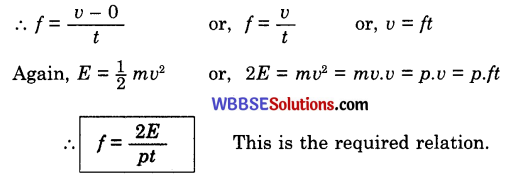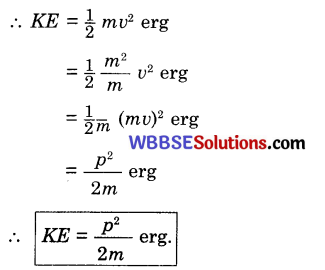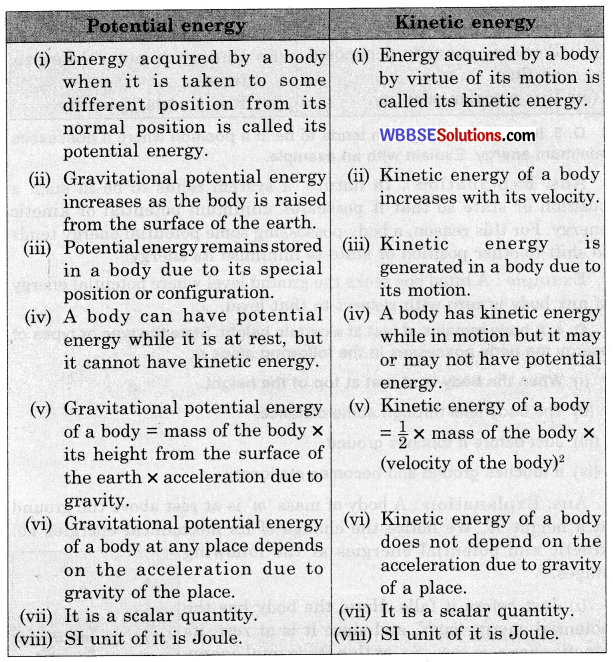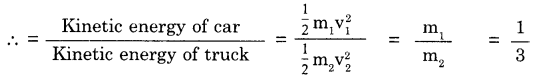# WBBSE Class 9 Physical Science Solutions Chapter 5 work, power and energy

Detailed explanations in West Bengal Board Class 9 Physical Science Book Solutions Chapter 5 work, power and energy offer valuable context and analysis.

## WBBSE Class 9 Physical Science Chapter 5 Question Answer – Chapter 5 Work, Power and Energy

Question 1.
What is the absolute unit of force in SI system?
1 joule.

Question 2.
What is power?
Work done in unit time is called power.Question 3.
What is the relation between power and force?
Power = force X velocity.

Question 4.
What is energy of a body?
Energy is defined as the capacity of a body to perform work.

Question 5.
Is work a scalar or a vector quantity?
Work is a scalar quantity.

Question 6.
Write down the absolute and gravitational SI units of work.
The absolute SI unit of work : joule.
The gravitational SI unit of work : kg-m.

Question 7.
What is the relation between work and power?
Work done (W) = Power P) x Time (t)Question 8.
What is the gravitational SI unit of power?
The gravitational SI unit of power: kg-m/sec

Question 9.
How many kinds of mechanical energy are there?
Mechanical energy is of two types :

• Potential energy and
• Kinetic energy.

Question 10.
How is work measured?
Work done (W = Force (F) x Displacement (S)

Question 11.
What is the practical unit of work?
The practical unit of work is Joule.

Question 12.
Give the expression of kinetic energy ?
Kinetic energy =$$\frac{1}{2}$$ × mass × (velocity)2

Question 13.
Give the expression of gravitational potential energy ?
Gravitational potential energy = mass of the body (m) x acceleration due to gravity (g) x height of the body above the surface of the earth (h).Question 14.
A man swims in a river against the current water. If he remains fixed with respect to shore, does he work with respect to shore ?
Here work done by the man is zero as his displacement with respect to the shore is zero.

Question 15.
Define watt.
If an agent does 1 joule of work in 1 second, its power is 1 watt.

Question 16.
Define horse power.
The power of work at the rate of 550 ft-lb per second is called one horse power.

Question 17.
Does applied force always perform work ?
No work force :

• If there is no displacement of the point of application of the force.
• Again if any force acts perpendicularly to the direction of the displacement.

Question 18.
Is work done due to rotation of the earth round the sun ?
A force does no work in a direction perpendicular to its direction of application. Here no work is done.

Question 19.
What do you mean by the rate of work done ?
Power.

Question 20.
What is the relation between horse power and watt ?
1 horse power = 746 watt.Question 21.
Give an example of a scalar quantity where three fundamental units are used.
Power

Question 22.
Is energy a scalar or a vector quantity ?
Energy is a scalar quantity.

Question 23.
What is the SI unit of power ?
Watt.Question 24.
What is the SI unit of energy ?
Joule

Question 25.
A heavier body and a lighter body are moving with the same velocity. Which of them can do more work ?
Heavier body can do work more.

Question 26.
How much work is done by a man who tries to push the wall of a house but fails to do so.
No work is done in this case.

Question 27.
What is the work done by the earth in moving around the Sun ?
No work is done.

Question 28.
When is work done by a force negative ?
The work done is negative when force acts opposite to the direction of the displacement.

Question 29.
When is work done by a force positive ?
The positive work is done when the displacement is in the direction of the force.

Question 30.
identify the kind of energy possessed by a rolling stone.
Kinetic energy.Question 31.
State the law of conservation of energy.
Law of conservation of energy: When one form of energy is changed into other forms of energy, the total energy of an isolated system remains the same i.e. the total energy before transformation is equal to the sum of the different energies transformed.

Question 32.
Define Potential energy.
Potential energy – Energy acquired by a body by virtue of its position or configuration is called its potential energy and measured by the amount of work the body can do in passing from its present position or configuration to some standard position or configuration.

Question 1.
Define work.
Work : Work is said to be done when the point of application of a force moves and is measured by the product of the force and the distance moved in the direction of the force.

Question 2.
How is work measured?
Measurement of work:• If the force F acts on a body and causes a displacement S, then the amount of work done (w) F × S,
• If the direction of displacement makes an angle θ with the direction of the applied force, then component of the force along the direction of displacement will he F cos θ.
So, work done (w) = F cosθ.S = FS cosθ.Question 3.
Will work be done by applied forces in all cases ?
No work force :

• If there is no displacement of the point of application of the force, then the force is called a no work force.
• Again, if any force acts perpendicularly to the direction of the
displacement, then the force does not do any work. Because in this case 0 90° and cos 90° = 0.
The amount of work done = F cosθ.S = F cos 90°.S = 0.

Question 4.
Show with an example that work is done by overcoming some resistive force.
When a body lying on a surface is pushed, it moves overcoming the opposing frictional force. Here the body when moves, does some work by overcoming frictional force offered by the surface.

Question 5.
What do you understand by ‘work done by a force’ ? Give one example.
Work done by a force : If the displacement of the point of application of the force takes place in the direction of the force then the force is said to have done the work.
Example : When a body is dropped vertically the gravitational force attracts it downward. The displacement of the body takes place in the direction of gravitational force. Here work is done by the gravitational force.

Question 6.
What do you understand by ‘work done against a force’? Give one example.
Work done against a force: When the displacement of the point of application of the force takes place in the direction opposite to the applied force, then work is said to be done against the force.
Example: When a body is lifted vertically upward, displacement of the body is in the direction opposite to the gravitational force and work is done against the gravitational force.

Question 7.
What is no-work force ? Give an example.
No-work force: When a force acts perpendicular to the direction of displacement of a particle, then the force does not do any work and is known as no-work force.
Example: A porter while carrying luggage does no work, since, he moves horizontally and perpendicular to the force of gravity. The porter, of course, did work at the beginning while he lifted the luggage against the gravity.Question 8.
State an unbalanced force and indicate the direction and the work it does.
Two unequal oppositely directed forces acting on a body create an unbalanced force which is equal to the difference of the forces. The unbalanced force causes displacement and work on the body in the direction of the greater force.

Question 9.
Deduce the relation between joule and erg.
Relation between joule and erg :
1 joule = 1 Newton × 1 meter
= 105 dyne × 100 cm
= (105 × 102) dyne-cm
= 107 erg
∴ 1 joule = 107 erg

Question 10.
Are watt and watt-hour same physical quantities ? Explain.
Explanation: Watt and watt-hour are not same physical quantities. Watt which means 1 joule/sec is the unit of power, i.e. work done per unit time, but watt-hour (work/time x time) which equals to work or energy. 1 watt-hour is the work performed by a machine or an agent which works at the rate of 1 joule per second.

Question 11.
Establish a relation between power, force and velocity.
Relation between power, force and velocity
$$\text { Power }=\frac{\text { work }}{\text { time }}$$∴ Power = Force × velocity

Question 12.
If an agent does large amount of work, does it always mean that the agent possesses large power ?
Explanation: Only the magnitude of work performed does not clearly indicate the power of a body, if the time in which the work performed is mentioned. It gives the idea of power clearly.

A large quantity of work done, but in a long time, results to a small power but a smaller quantity of work done in a very short period of time results to a greater power.Question 13.
Express kinetic energy of a body in terms of momentum and velocity.
Kinetic energy : Kinetic energy is the energy which a body possesses by reason of its motion.
Kinetic energy (KE) – $$\frac{1}{2}$$ × m × υ2
(m = mass of the body
υ = velocity of the moving body)
∴ KE = $$\frac{1}{2}$$m×υ×υ2
(m = mass of the body
υ = velocity of the moving body)
∴ KE = $$\frac{1}{2}$$mυ.υ
$$\frac{1}{2}$$p.υ
(p = mυ = momentum of the body)
So, Kinetic energy = $$\frac{1}{2}$$ × momentum × velocity

Question 14.
A particle starting from rest requires kinetic energy ‘E’ in time ‘f and its momentum is ‘p’. Find Its acceleration In terms of E, p and t
Deduction: Let acceleration be f, velocity acquired in time t be y and mass of the body be m.Question 15.
Find the kinetic energy (KE) of a particle of mass ‘m’ gram and momentum ‘p’ g-cm/sec in terms of ‘m’ and ‘p’.
Deduction: Let the particle moves with velocity υ cm/sec.This is the required relation.Question 16.
Momentum of a heavy body is equal to that of a light body. Which of the two possesses greater kinetic energy?
Explanation: Let the mass of the heavy body be M and its velocity υ. The corresponding quantities of the light body are
and ‘υ’.
So, by the given condition, MV = mυ. Since, M is greater than ‘m’,
so ‘υ’ must be proportionally greater than V, for keeping equality between MV and mυ.
Now, if mυ be multiplied with the larger quantity υ and MV with smaller quantity V, the first product mυ.υ will be larger than the second product MV V.
Hence, m.υ.υ. > MV.V.
or, $$\text { or, } \frac{1}{2} m v^2>\frac{1}{2} M V^2$$
i.e. kinetic energy of the light body> kinetic energy of the heavy body.

Question 17.
Two bodies of unequal masses have the same kinetic energy. How do their momenta compare in terms of their masses?
Explanation: Let masses of the bodies be m1, m2 and their corresponding velocities be υ1 and υ2.Thus, momenta of the two bodies are directly proportional to the square root of their corresponding masses.

Question 18.
A heavy body and a light body have equal kinetic energy, which of them possesses greater momentum?
Explanation: Let the mass of a heavier body be m1 and that of the lighter body be m2 also let the velocity of the heavier body be y1 and that of the lighter body be y2.So, momentum of the heavier body (m1υ1) is greater than the momentum of the lighter body (m2υ2)

Question 19.
A man carrying a bag stands in an ascending lift. Explain
(i) does the man do work on the bag?
(ii) is there any change of energy occuring in the bag?
Explanation:
(i) Since the weight does not displace with respect to the man, so the man does no work on it.
(ii) The height of the weight from ground gradually increases, so, its potential energy increases. Also, the weight moves upward with the same velocity as that of the lift, so it has kinetic energy.

Question 20.
What is potential energy? Write down a mathematical expression for potential energy of a certain mass raised to a certain height above ground.
Potential energy: Potential energy is the energy which a body possesses by virtue of its position or configuration.
Calculation: Weight of the body of mass ‘m’ is mg, where ‘g’ is acceleration due to gravity at the place. To raise the body, an upward force mg has to be applied on it. If it is raised ‘h’ distance above,
work done = mg x h [Force x displacement]
∴ work done = mgh
This work remains stored in the body as potential energy.Question 21.
Is work done when the Earth moves round the Sun ?
Explanation: The centripetal force (the force with which the sun attracts the earth) of the Sun on the Earth does no work, since direction of displacement of the Earth at every point of its orbit is perpendicular to it. So, it is a no-work force.

Question 22.
In a tug of war one team wins over the other. Which team does work and how ?
Explanation: In a tug of war the winning team works against the force applied by the losing team who are displaced through some distance.

Question 23.
A moving object suddenly comes to rest. Which energy converts to which other in this case ?
In moving condition the body possesses kinetic energy. When it stops, the kinetic energy is expended in doing mechanical work against the opposing forces which stopped the body.

Question 24.
Which energy converts to which other when the spring in a toy is compressed ?
Explanation: While the spring of a toy is brought to a compressed condition mechanical energy is expended. This energy remains stored in the spring as potential energy created due to change of configuration of different parts of the spring.

Question 25.
A man carrying a briefcase in hand walks on flat horizontal surface. Does he do work ?
Reason: The man does not do work on the briefcase. Because, as the man holds the briefcase in hand, he exerts a force on it vertically upward but the briefcase is made to move horizontally. So, the force and its point of application are perpendicular to each other, i.e. no work is done.

Question 1.
What is the comparison of potential and kinetic energy?
Comparison of potential and kinetic energy :Question 2.
What is the comparison of energy and power?
Comparison of energy and power:

 Energy Power (i) The capacity of a body or person to do work is its energy. (i) Power is the rate of doing work. (ii) Energy of two persons may be same, but their powers may be different. (ii) The quantity of energy of two persons may be different, although they have same power. (iii) Power is quantity dependent on time. (iii) Energy is not related to time. (iv) It is a scalar quantity. (iv) It is a scalar quantity.

Question 3.
In nature, any system tends to be in a position where it possesses minimum energy. Explain with an example.
Explanation: In nature, a system tends to be in such a position or state so that it possesses minimum potential or kinetic energy. For this reason, a body possessing some potential energy tends to shift to other position or state to minimise its energy.
Example: A lifted boy seeks the ground level where potential energy of any body is zero with respect to that level.Question 4.
A body remains at rest at a certain height. State the type or types of energy the body possesses in the following steps :
(i) When the body is at rest at top of the height.
(ii) The body falls through some distance.
(iii) Just before it touches ground.
(iv) it touches ground and becomes stationary.
Explanation : A body of mass ‘m’ is at rest above the ground at a height ‘h’. We notice the change of its mechanical energies i.e. kinetic and potential energies at the following stages.

(i) Just before it falls. Here, the body has the potential energy ‘mgh’, and since it is at rest, its kinetic energy is zero. So, at this stage total energy of the body is mgh.

(ii) The body descends through ‘a’, where, a < h.
The height of the body above ground is (h – a), so its potential energy is mg (h – α). The potential energy thus decreases by mga. While the body descends, it acquires some velocity, υ(say). So its kinetic energy is $$\frac{1}{2}$$ mυ2 which can be proved equal to mga. Thus decrease of potential energy equals gain in kinetic energy.
So, the total energy
= mg (h – α) + mga
= mgh – mgα + mgα = mgh

(iii) The body is just above the ground before touching it. Here ‘h’ is almost zero, so the potential energy is negligible. The body possesses only kinetic energy which may be proved equal to mgh. Thus, at all stages, the total energy of the body, before it touches ground, is constant and equal to mgh.

(iv) When the body touches ground ‘h’ is zero, so potential energy is zero. Since, it comes to rest, its kinetic energy is also zero. The energy of the body dissipates in environment as heat energy and sound energy.

Question 5.
Can a body possess energy without momentum ? is the reverse possible ?
Explanation: A body remaining motionless at a certain position other than its normal position on the surface of the earth may have potential energy but it is without momentum. The body also has no kinetic energy.

In the reverse case, if the body has momentum it must have velocity and thus kinetic energy, hence momentum without kinetic energy is not possible. Of course, the body may have momentum without potential energy, if it confines its motion on the surface of the earth.

Numerical Problems

Working formula

(i) Work done = Force x displacement of the point of application.

(ii) Power (p) = $$\frac{\text { work done }(w)}{\text { time taken }(t)}$$

(iii) Kinetic energy (KE) = $$\frac{1}{2}$$ × mass of the body (m) × (velocity of the body)2 (y)2

(iv) Potential energy
(PE) – mass of the body (m) × acceleration due to gravity (g) × height of the body above the surface of the earth (h)

(iv) 1 Horse power = 746 watt

(vi) 1 kilowatt = 103 watt

(vii) 1 mega watt = 105 watt.

Problem 1.
A rifle bullet of mass 15g travelling at a speed of 300 meter/sec. Find the kinetic energy of the bullet
m = 15, g = 0.015kg
y = 300 m/sec
KE =?
kinetic energy = $$\frac{1}{2}$$ × mass ×(velocity)2
= $$\frac{1}{2}$$ × m × ν2
= $$\frac{1}{2}$$ × 0.015 x (300)2
= 675 joule.Problem 2.
The power of a motor car is 10 kW. What is the amount of work done by the car in 1 hr?
1 hr = 60 × 60 = 3600 sec
1 kW = 103W
Power of the car = 10 kW = 10 × 103 J/sec
∴ work done by the car in 1 hr
= 10 × 103 x 3600J
= 36 × 107J

Problem 3.
If a pump lifts 500 liter of water per hour to a height of 30m. Then find the work done by the pump and its power in watt. [mass of 1 liter of water = 1 kg]
Work done by the pump
= weight of water x displacement of water
= 5000 × 1 × 98 × 30J
= 14.7 × 105J
Power of the pump = $$\frac{\text { Work done }}{\text { time }}=\frac{14.7 \times 10^5 \mathrm{~J}}{60 \times 60 \mathrm{sec}}$$
= 408W

Problem 4.
If a boy weighing 40 kg runs up a flight of stairs to a height of 10 m against the gravitational pull on him, i.e. his weight, so
Work done = 40 × 98 × 10 = 3920 J
Power of the boy = $$\frac{\text { Work done }}{\text { Time }}=\frac{3920}{15}$$ = 261.33W

Problem 5.
If 150 joule work is done for the displacement of 15 m of a body. Calculate the amount of force applied here.
Work is done here = 150 joule
displacement = 50 m
we known,Applied force  = $$\frac{\text { Work done }}{\text { displacement }}$$
= $$\frac{150}{50}$$ = 3 NewtonProblem 6 :
If 10 Newton force is applied on a body then 5 m displacement of the body occurs towards the direction of the force. What will be the work done?
We know,
Work done = Force x displacement
= 10 × 5 Newton-meter
= 50 joule

Problem 7.
If 100 g mass of a body is dropped from 80 cm high place then calculate the amount of potential energy is lost during this
process.
m = 100 g = 0.1 kg
g = 98 m/sec2
h = 80 cm = 0.8 m
Amount of potential energy lost = m × g × h
= 0.1 × 9.8 × 0.8 joule
= 0.784 joule

Problem 8.
One body has mass 50 g and velocity 10 cm/sec. The other body has mass 20 g and velocity 20 cm/sec. Compare their velocities and kinetic energies.
We know,
Momentum of a body = mass of the body × velocity
Here, velocity of first body : velocity of second body
=(50×10):(20×20) = 5 : 4
We also know,
Kinetic energy = $$\frac{1}{2}$$ × mass × (velocity)2
Here, kinetic energy of first body: kinetic energy of second body
= $$\frac{1}{2}$$× 50 × (10)2 : $$\frac{1}{2}$$ × 20 × (20)2
∴ The ratio of velocities of two bodies = 5 : 4
The ratio of kinetic energies of two bodies 5 : 8

Problem 9.
A rifle bullet of mass 50 g travelling at a speed of 200 rn/sec. Find the kinetic energy of the bullet.
m= 50g g = 0.05kg
υ = 200 m/sec
KE=?
Kinetic energy = $$\frac{1}{2}$$ × mass × (velocity)2
$$\frac{1}{2}$$ × m × (velocity)2
= $$\frac{1}{2}$$ × m x ν2
= $$\frac{1}{2}$$ 005 × (200)2
= 103 jouleProblem 10.
The power of a machine is 40 W. What is the amount of work done by the machine in 30 sec?
Power of the machine = 40 W
= 40 joule/sec
∴ Work done by the machine (40 × 30) joule
= 1200 joule

Problem 11.
A car and a truck have the same speed of 30ms. If their masses are in the ratio of 1: 3, find the ratio of their kinetic energies.